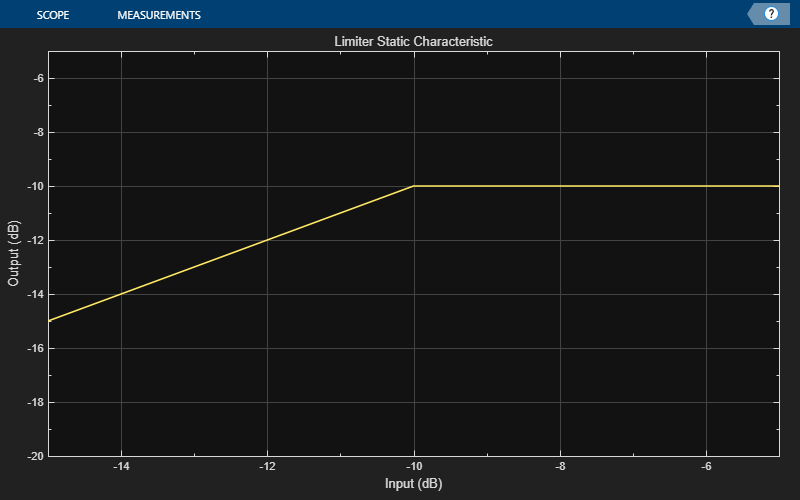# staticCharacteristic

Return static characteristic of dynamic range controller

## Syntax

``outputLevel = staticCharacteristic(dynamicRangeController)``
``outputLevel = staticCharacteristic(dynamicRangeController,inputRange)``

## Description

example

````outputLevel = staticCharacteristic(dynamicRangeController)` returns the static characteristic of the dynamic range control object.```

example

````outputLevel = staticCharacteristic(dynamicRangeController,inputRange)` enables you to specify the input range.```

## Examples

collapse all

Create a `limiter` System object™. Get the output level of the static characteristic over a specified range.

```dynamicRangeLimiter = limiter; inputLevel = -15:1:-5```
```inputLevel = 1×11 -15 -14 -13 -12 -11 -10 -9 -8 -7 -6 -5 ```
`outputLevel = staticCharacteristic(dynamicRangeLimiter,inputLevel)`
```outputLevel = 1×11 -15 -14 -13 -12 -11 -10 -10 -10 -10 -10 -10 ```

Plot the static characteristic. Modify the title to state that the object is a limiter.

```hvsz = visualize(dynamicRangeLimiter,inputLevel); hvsz.Title = "Limiter Static Characteristic";```## Input Arguments

collapse all

Dynamic range control object, specified as a `compressor`, `expander`, or `limiter` System object™.

Range over which to calculate the output of the static characteristic, specified as a vector of monotonically increasing values expressed in dB. The default input range is `[-50:0.01:0]` dB.

## Output Arguments

collapse all

Output level in dB, returned as a vector the same size as `inputRange`.

## Version History

Introduced in R2022a